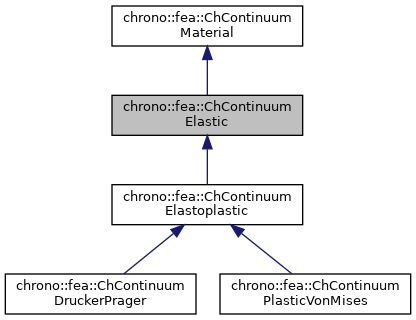chrono::fea::ChContinuumElastic Class Reference

## Description

Class for the basic properties of materials in an elastic continuum.

This is a base material with isotropic hookean elasticity.

#include <ChContinuumMaterial.h>

Inheritance diagram for chrono::fea::ChContinuumElastic:[legend]
Collaboration diagram for chrono::fea::ChContinuumElastic:[legend]

## Public Member Functions

ChContinuumElastic (double myoung=10000000, double mpoisson=0.4, double mdensity=1000)
Create a continuum isotropic hookean material. More...

ChContinuumElastic (const ChContinuumElastic &other)

void Set_E (double m_E)
Set the Young E elastic modulus, in Pa (N/m^2), as the ratio of the uniaxial stress over the uniaxial strain, for hookean materials. More...

double Get_E () const
Get the Young E elastic modulus, in Pa (N/m^2).

void Set_v (double m_v)
Set the Poisson v ratio, as v=-transverse_strain/axial_strain, so takes into account the 'squeezing' effect of materials that are pulled (so, if zero, when you push the two sizes of a cube, it won't inflate). More...

double Get_v () const
Get the Young v ratio, as v=-transverse_strain/axial_strain.

void Set_G (double m_G)
Set the shear modulus G, in Pa (N/m^2), as the ratio of shear stress to the shear strain. More...

double Get_G () const
Get the shear modulus G, in Pa (N/m^2)

double Get_l () const
Get Lamé first parameter (the second is shear modulus, so Get_G() )

double Get_BulkModulus () const
Get bulk modulus (increase of pressure for decrease of volume), in Pa.

double Get_WaveModulus () const
Get P-wave modulus (if V=speed of propagation of a P-wave, then (M/density)=V^2 )

void ComputeStressStrainMatrix ()
Computes Elasticity matrix and stores the value in this->StressStrainMatrix Note: is performed every time you change a material parameter.

ChMatrixDynamicGet_StressStrainMatrix ()
Get the Elasticity matrix.

void ComputeElasticStress (ChStressTensor<> &mstress, const ChStrainTensor<> &mstrain) const
Compute elastic stress from elastic strain (using column tensors, in Voight notation)

void ComputeElasticStrain (ChStrainTensor<> &mstrain, const ChStressTensor<> &mstress) const
Compute elastic strain from elastic stress (using column tensors, in Voight notation)

void Set_RayleighDampingM (double m_d)
Set the Rayleigh mass-proportional damping factor alpha, to build damping R as R=alpha*M + beta*K.

double Get_RayleighDampingM () const
Set the Rayleigh mass-proportional damping factor alpha, in R=alpha*M + beta*K.

void Set_RayleighDampingK (double m_d)
Set the Rayleigh stiffness-proportional damping factor beta, to build damping R as R=alpha*M + beta*K.

double Get_RayleighDampingK () const
Set the Rayleigh stiffness-proportional damping factor beta, in R=alpha*M + beta*K.

virtual void ArchiveOut (ChArchiveOut &marchive) override

virtual void ArchiveIn (ChArchiveIn &marchive) overridePublic Member Functions inherited from chrono::fea::ChContinuumMaterial
ChContinuumMaterial (double mdensity=1000)

ChContinuumMaterial (const ChContinuumMaterial &other)

void Set_density (double m_density)
Set the density of the material, in kg/m^2.

double Get_density () const
Get the density of the material, in kg/m^2.Protected Attributes inherited from chrono::fea::ChContinuumMaterial
double density

## ◆ ChContinuumElastic()

 chrono::fea::ChContinuumElastic::ChContinuumElastic ( double myoung = 10000000, double mpoisson = 0.4, double mdensity = 1000 )

Create a continuum isotropic hookean material.

Default value for Young elastic modulus is low (like a rubber-type material), and same for density.

## ◆ Set_E()

 void chrono::fea::ChContinuumElastic::Set_E ( double m_E )

Set the Young E elastic modulus, in Pa (N/m^2), as the ratio of the uniaxial stress over the uniaxial strain, for hookean materials.

Intuitively, the tensile pressure on a side of a parallelepiped in order to double its length. Note that most metal materials require very high values, ex. steel has E=210GPa (E=210e9), aluminium E=69e9, and this can cause numerical problems if you do not set up the simulation integrator properly.

## ◆ Set_G()

 void chrono::fea::ChContinuumElastic::Set_G ( double m_G )

Set the shear modulus G, in Pa (N/m^2), as the ratio of shear stress to the shear strain.

Setting G also changes Poisson ratio v.

## ◆ Set_v()

 void chrono::fea::ChContinuumElastic::Set_v ( double m_v )

Set the Poisson v ratio, as v=-transverse_strain/axial_strain, so takes into account the 'squeezing' effect of materials that are pulled (so, if zero, when you push the two sizes of a cube, it won't inflate).

Most materials have some 0<v<0.5, for example steel has v=0.27..0.30, aluminium v=0.33, rubber=0.49, etc. Note! v=0.5 means perfectly incompressible material, that could give problems with some type of solvers. Setting v also changes G.

The documentation for this class was generated from the following files:
• /builds/uwsbel/chrono/src/chrono/fea/ChContinuumMaterial.h
• /builds/uwsbel/chrono/src/chrono/fea/ChContinuumMaterial.cpp